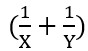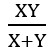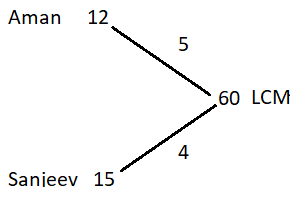# Time and work – Concepts and Formula

## Time and work concpets

One of the most prevalent quantitative aptitude topics asked in government tests is Time and work. This is one of those topics that students are already aware of before they begin studying for competitive exams.

Although the concepts of Time and work remain the same, the kind of questions presented in exams may vary.

2-3 word problems related to Time and work are asked in exams, but candidates may also expect questions about data sufficiency and data interpretation related to Time and work.

So, we are providing you with concepts and formulas of Time and work.

### Basic concept of Time and work

Time: Time is the duration during which any activity or work happens or continues.

Work: Work is a task or set of activities to achieve a certain result.

If a person A completes a work in X days, then the amount of work completed by him in 1 day will be = 1/X

Eg. If Ram can complete a work in 20 days, then work done by Ram in one day is  1/20

Or If a person A completes  1/X part of a work in one day then, it will take X days to complete the whole work.

Eg.If Manoj complete 1/3 of work in one day then, he can complete the whole work in 3 days.
If Laxman can complete  2/3 of work in one day, then he can complete the whole work  in 3/2 (1.5 days)

Similarly, if a person B completes a work in Y days, then the amount of work completed by him in 1 day will be = 1/Y

We can say that in one day A and B together can completeamount of work. Thus together A and B can compelte the work inExample 1 : Time taken by Aman and Sanjeev alone to complete a piece of work is 12 days and 15 days, respectively. What is the time taken by Aman and Sanjeev together to complete the work?

Solutions

Aman complete the whole work in 12 days
Work done by Aman in one day = 1/12
Sanjeev complete the whole work in 15 days
Work done by Sanjeev in one day = 1/15
One day work of Aman and SanjeevSo, Aman and Sanjeev can do 3/20 part of the in one day
So, time taken by Aman and Sanjeev together to complete the work will be
= 20/3 = 6(2/3) days

## Alternative Method: LCM method

We will take the same example to explain the LCM method

Example: Time taken by Aman and Sanjeev alone to complete the a piece of work is 12 days and 15 days, respectively. What is the time taken by Aman and Sanjeev together to complete the work?

Step 1: Calculate total work
In this LCM method, we will assume the total work be LCM of 12 and 15 = 60 units

Step 2 : Calculate the efficiency of each individual

Efficiency = One day work

Efficiency = Total Work/ Total Time

Efficiency of Aman = 60/12 = 5

Efficiency of Sanjeev = 60/15 = 4So, Efficiency of Aman and Sanjeev = 5+4 = 9
Or we can say, Work done by Aman and Sanjeev in one day = 5+4 = 9 units

Time taken by Aman and Sanjeev

= Total work / Efficiency

= 60/9 = 20/3 = 6(2/3) days

### Solved problems on time and work

Question 1 : Rohit is twice as efficient as Sapna and can finish a piece of work in 25 days less than Sapna. Sapna can finish this work in how many days?

Solutions : Efficiency of Rohit  : Efficiency of Sapna = 2 : 1

So, ratio of Time taken by Rohit: Time taken by Sapna = 1 : 2

Let time taken by Rohit is x and Sapna is 2x

Then, According to Questions

2x – x = 25

x = 25

2x = 50,

So, Sapna takes 50 days to complete the work

Question 2 Chanchal is thrice as efficient as Mohan and together they can finish a piece of work in 60 days. Mohan will take how many days to finish this work alone?

Solutions :  Efficiency of Chanchal  : Efficiency of Mohan = 3 : 1

Let one day work of Chanchal and Mohan is 3x and x respectively

Total work  = Efficiency x time = ( 3x + x ). 60 = 240x

Time taken by Mohan to finish the work  = Total work / Efficiency = 240x / x = 240

### Relation between Time and Efficiency

Efficiency is inversely proportional to the time.

So, If A is more efficient than B,  then A will take less time to the same work

Example. If the efficiency of A is twice that B, then time taken by B is twice of time taken by A

If the ratio of efficiency of A and B is x : y , then ratio of time taken by A and B will be y : x

Question 3 Efficiency of  Mohit is 20% more than Vandana. If Mohit takes 10 days to complete the work, then find the time taken by Vandana to complete the same work.

Solutions Let the efficiency of Vandana is 100x
So, efficiency of Mohit is 120x

Ratio of efficiency of mohit : efficiency of Vandana = 120x : 100x = 6:5

Ratio of time taken by mohit and Vandana will be  5 : 6

Time taken by Vandana = 10/5  x 6 = 12 days